# Relative velocities and the speed of light

ViolentCorpse
Hello everyone,

One of the conclusions of relativity is that no massive object can move at v ≥ c, but with respect to what, I don't understand? Let me try making my question clearer with an example:

Suppose there are two bodies both moving side by side at v=0.9c relative to earth. The relative speed between the two bodies is 0. So I wonder, is it possible for one of the bodies to accelerate to 0.1c or more with respect to the other body? It would seem perfectly possible as long as the moving bodies are all we're looking at, but there's also earth to consider, relative to which, the speed of the moving body was already 0.9c. So I'm a little confused; would that body be able to accelerate to 0.1c relative to the other body making the relative velocity between it and the earth equal to 1c? I suppose not, but considering that some basic equations of special relativity, like time dilation, have only the relative velocity variable in them between the two moving frames in question... it seems, at first glance, that it just might be possible...

So I could definitely use some help here, lol.

Thank you!Last edited:

## Answers and Replies

Mentor
would that body be able to accelerate to 0.1c relative to the other body

Yes, but that doesn't mean that this...

making the relative velocity between it and the earth equal to 1c?

...will happen. Check out the correct relativistic velocity addition formula:

•1 person
Velocities don't add that way. If one body sees the other accelerate to velocity u, and both bodies were initially doing velocity v relative to the earth, then an observer on earth will see the accelerated body doing $$u'=\frac{u+v}{1+uv/c^2}$$In this case, your accelerated ship will be doing c/1.09.

This is, in fact, always true. If I see two cars going in opposite directions at 60mph, the drivers of the cars will see the other approaching them at very slightly less than 120mph. However, they'll need extremely precise measurements to see it - in my head I make it something like 119.999999999999mph.

•1 person
ViolentCorpse
Ohh. I knew about the relativistic velocity addition formula, but for some odd reason I couldn't get myself to apply it to this problem (I obviously didn't understand it very well).

I understand it now. Thank you so much, PeterDonis and Ibix!

Gold Member
Hello everyone,

One of the conclusions of relativity is that no massive object can move at v ≥ c, but with respect to what, I don't understand? Let me try making my question clearer with an example:

Suppose there are two bodies both moving side by side at v=0.9c relative to earth. The relative speed between the two bodies is 0. So I wonder, is it possible for one of the bodies to accelerate to 0.1c or more with respect to the other body?
The easiest way I know to illustrate this is in the initial rest frame of the two bodies so that one of them can accelerate away to 0.1c from the first one while the earth is moving away in the opposite direction at -0.9c. Here is a spacetime diagram to depict this. Earth is in blue, the first body is in black and the second one is in red. The dots represent one-second intervals of time for each body: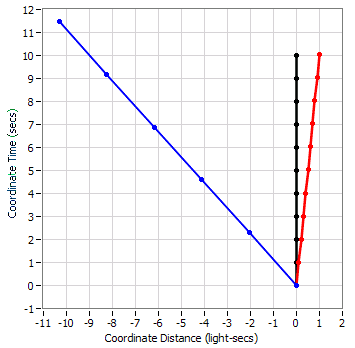Now you can easily see that at a Coordinate Time of 10 seconds, there is exactly 10 light-seconds of distance between earth and the second body.

It would seem perfectly possible as long as the moving bodies are all we're looking at, but there's also earth to consider, relative to which, the speed of the moving body was already 0.9c. So I'm a little confused; would that body be able to accelerate to 0.1c relative to the other body making the relative velocity between it and the earth equal to 1c?
To see the relative velocity between the earth and the second body, we need to transform the coordinates of the events (the dots) to the rest frame of the earth: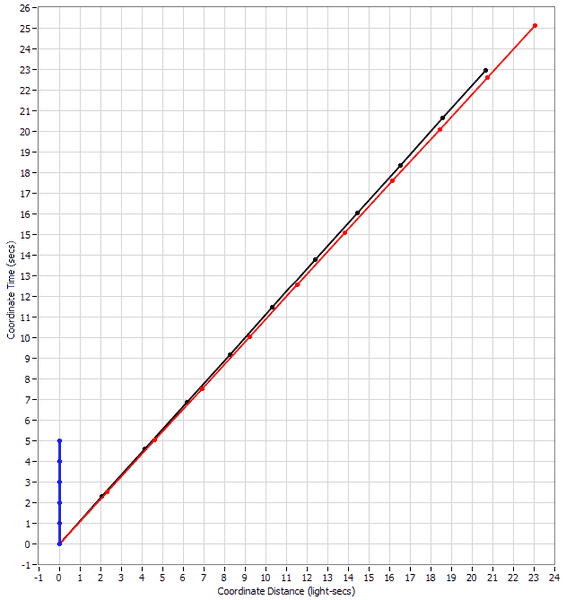Now you can see that the speed of the second body, in the rest frame of earth is not c but something less than c. In fact if we look at the Coordinate Time of 24 seconds, we see that the body is at a distance of 22 light-seconds from earth so its speed is 22/24 c which equals 0.917c. As Ibix pointed out in post #3, the speed according to the formula is c/1.09 which also comes out to be 0.917c.

I suppose not, but considering that some basic equations of special relativity, like time dilation, have only the relative velocity variable in them between the two moving frames in question... it seems, at first glance, that it just might be possible...

So I could definitely use some help here, lol.
The equation for Time Dilation refers not to relative velocities between two frames (or two bodies) but rather the speed of a single body according to a single reference frame. It refers to the ratio of an interval of a reference frame's Coordinate Time to the same interval for a body's time. Note in the above two diagrams that the relative speed between the earth and the first (black) body is the same, 0.9c, but they have different Time Dilations because they have different speeds in the two frames. In the first diagram, the earth has a speed of 0.9c which gives a Time Dilation factor of 2.294 so its one-second intervals marked by the dots are vertically spaced 2.294 times the grid line spacings for the Coordinate Time. Similarly, in the second diagram, the first (black) body's dots are spaced farther apart than the grid lines.

Thank you!You're welcome. Any more questions?

#### Attachments

Last edited:
•1 person
ViolentCorpse
Thank you for such a detailed answer, ghwellsjr! Much appreciated!There's one more thing I'd like to ask: Do time dilation and length contraction occur in order to prevent any massive object from reaching c? For example, if an observer in frame 1 wants to measure the speed of frame 2 (which is moving relativistically fast) would the speed measurements that he'd get be smaller than they would've been had there been no time dilation effect?

Homework Helper
A fundamental point of relativity is that the speed of light is the same relative to all observers so the speed of light is the one speed for which you do not need to specify a reference frame. It is impossible for an object to move faster than the speed of light relative to any observer.

Gold Member
Thank you for such a detailed answer, ghwellsjr! Much appreciated!There's one more thing I'd like to ask: Do time dilation and length contraction occur in order to prevent any massive object from reaching c? For example, if an observer in frame 1 wants to measure the speed of frame 2 (which is moving relativistically fast) would the speed measurements that he'd get be smaller than they would've been had there been no time dilation effect?
All observers are in all frames so I don't know what you mean by saying "an observer in frame 1". Do you mean an observer that is at rest in frame 1, like at the beginning of my previous response where I said that the two bodies were initially at rest? But note that the earth was also in that first frame and traveling at -0.9c and I had the second body accelerate at the origin to 0.1c. The second frame that I showed also had all three objects traveling at different speeds and I could have transformed the scenario into other frames where none of the objects were at rest. Relativity says that all frames are equally valid for the depiction and analyis of any scenario. They all contain exactly the same information so you must not think of any frame as being preferred, not even one in which an object is at rest.

Since that is true, then it should be obvious to you that no observer can have any awareness of any frame, let alone trying to measure the speed of one frame relative to another frame. And since Time Dilation and Length Contraction of each object are different in each frame, no observer can be aware of any Time Dilation or Length Contraction.

However, one observer can measure the speed of another object relative to himself by several different means but they all require an assumption, typically about the speed of light, and they all yield the same answers. My favorite is the radar method which I will show you now. It works just like a radar cop using either radar or laser beams bouncing off a moving object and determining its speed by assuming that the beams take the same amount of time to get to the object as their reflections take to get back to the cop. The radar gun takes several measurements (or continuous measurements) over a period of time and calculates the speed of the object knowing the speed of light.

So let's see how earth can measure the speed of the first black body. Here is the first diagram from my previous post with a radar signal emitted from earth, bouncing off the black body and returning back to earth. I have marked in the times that the signal was sent and received. Now what earth does is divide the sum of those two times by two and determines that to be the time of the measurement (even though in this frame it isn't). Then earth divides the difference of those two times by two and determines that to be the distance to the body (even though in this frame it isn't at the determined time). By repeating this process, the earth can determine where the body was relative to itself at two different times and from that calculate the average speed during the determined time interval. Now in our case, earth can rely on the crossing of the earth and the two bodies at the origin of the frames to supply one of the determinations of distance.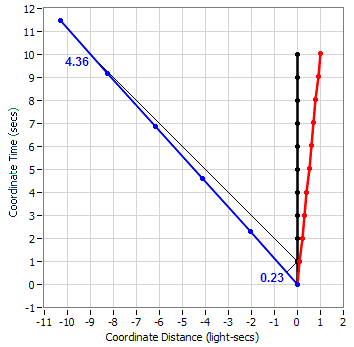Ok, let's see how this works. First, earth adds 0.23 and 4.36 to get 4.59 and dividing that by 2 yields 2.295 as the time of the measurement. Then the earth subtracts 0.23 from 4.36 to get 4.13 and dividing that by two yields a distance of 2.065. Now since our first "reading" was taken when everything was at zero, we can simply divide our distance by time to get speed. This would be 2.065/2.295 which equals 0.9, the correct speed of the black body relative to the earth.

Now let's see what the earth's radar measurement looks like in its own rest frame: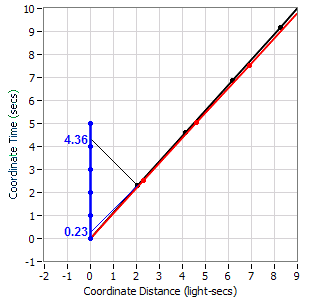Now we can see that the determinations that earth made match the coordinates in its own rest frame.

Now I will show you how the first black body can measure the speed of earth relative to itself: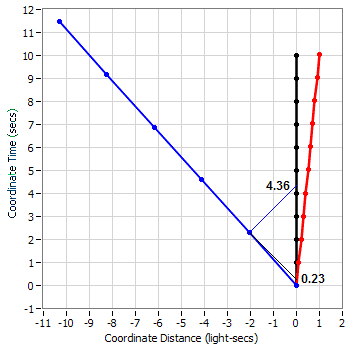Since these numbers are the same as for earth's case, the black body will determine that earth is moving away from it at 0.9c. I did this in the black body's rest frame but I could have done it in any other frame and the result would be identical.

Now it's time to answer your first question:

Do time dilation and length contraction occur in order to prevent any massive object from reaching c?

No. Time Dilation and Length Contraction are frame dependent (variant) effects and are the result of applying the Lorentz Transformation to the coordinates of events in one frame to get the coordinates of all the same events in a second frame moving with respect to the first one. It's the 1/(1-v2/c2) factor in the Lorentz Transformation that limits one frame from moving at c with respect to another frame and thus prevents any massive object from reaching c.

#### Attachments

•1 person
ViolentCorpse
Sorry for the poorly worded question. I should've been more exact.

Since that is true, then it should be obvious to you that no observer can have any awareness of any frame, let alone trying to measure the speed of one frame relative to another frame. And since Time Dilation and Length Contraction of each object are different in each frame, no observer can be aware of any Time Dilation or Length Contraction.
That's interesting. Some popular science texts I've read say things like "clocks moving with respect an observer's clock can be observed ticking slowly with respect to the latter" etc.

Thanks once again for the detailed explanation!nitsuj
Sorry for the poorly worded question. I should've been more exact.

That's interesting. Some popular science texts I've read say things like "clocks moving with respect an observer's clock can be observed ticking slowly with respect to the latter" etc.

Thanks once again for the detailed explanation!That is true, but surely the text also goes on to say how that is a symmetrical observation. That is the comparatively moving clock would observe the other clock as ticking more slowly. In other words both observe the Others clock as ticking more slowly.

The geometry of the each clocks observations is symmetrical, each has the same conclusion.

"clocks moving with respect an observer's clock can be observed ticking slowly with respect to the latter [and vice-versa]"

A clear example is, you right now are moving very fast compared to something "out there", but surely you don't notice any difference. It has no physical consequence to you, if something out there observes you at relativistic speeds here on Earth.

Proper time and proper length, comparative/relative motion and inertial are important terms to know.

Gold Member
Sorry for the poorly worded question. I should've been more exact.

Since that is true, then it should be obvious to you that no observer can have any awareness of any frame, let alone trying to measure the speed of one frame relative to another frame. And since Time Dilation and Length Contraction of each object are different in each frame, no observer can be aware of any Time Dilation or Length Contraction.

That's interesting. Some popular science texts I've read say things like "clocks moving with respect an observer's clock can be observed ticking slowly with respect to the latter" etc.

Thanks once again for the detailed explanation!I'm for full disclosure. Explain everything. Don't try to leave false impressions.

To me, the word "observe" refers to what you can see and just as it is possible for an observer to see a moving clock ticking more slowly than his own, he can at other times see that very same clock ticking more rapidly than his own. What these texts are referring to is what that observer can determine after performing radar measurements and logging them along with other oservations, assuming the same speed of light in both directions and doing a bunch of calculations.

Here is a spacetime diagram showing what the black body observes of the earth's clock before and after they pass: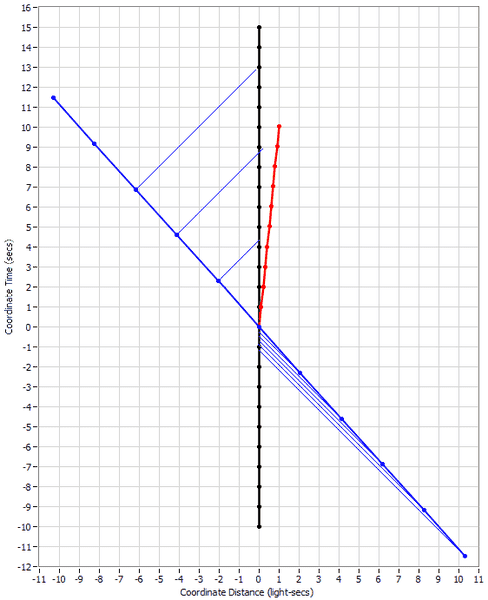The thin blue lines show the images of the earth's clock as they arrive at the black body. Prior to encounter, the body sees the earth's clock ticking much faster than its own and after encounter, much slower, even though the Time Dilation of the earth's clock in this frame is constant throughout and at a different factor than either of the observed tick rates.

Here's another spacetime diagram transformed such that both the black body's clock and the earth's clock are Time Dilated to the same extent: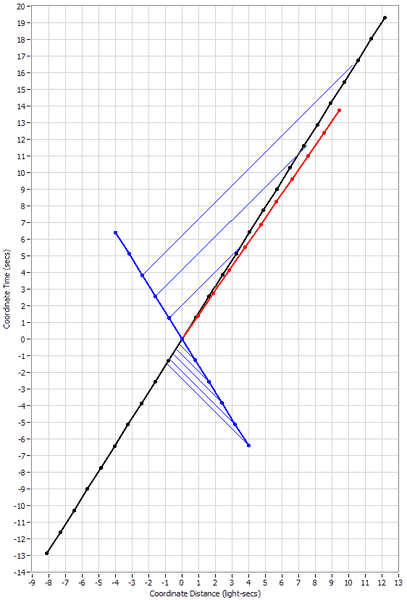Note that the observations don't change. Also note that I could have drawn in what the earth observes of the black body's clock and it would be a mirror image of the lines I did draw in showing that the observations are symmetrical.

If you're interested, I can show you how these observers can determine the Time Dilation using radar signals and making these same observations. That's what your texts are referring to.

#### Attachments

•1 person
nitsuj
I'm for full disclosure. Explain everything. Don't try to leave false impressions.

To me, the word "observe" refers to what you can see and just as it is possible for an observer to see a moving clock ticking more slowly than his own, he can at other times see that very same clock ticking more rapidly than his own.

Good point, my SR/GR pocket book defines "observation" as being measurements & calculations on the first few pages.

I didn't realize that was the point you are making.

ViolentCorpse
The thin blue lines show the images of the earth's clock as they arrive at the black body. Prior to encounter, the body sees the earth's clock ticking much faster than its own and after encounter, much slower, even though the Time Dilation of the earth's clock in this frame is constant throughout and at a different factor than either of the observed tick rates.

Oh wow. It never occurred to me that clocks could just as well be observed ticking faster than in the observer's inertial frame of reference. However, I don't understand the reason why the "ticking rate" is different before and after the encounter, even though the time dilation factor isn't changing?

Thank you soo much!

Mentor
I don't understand the reason why the "ticking rate" is different before and after the encounter, even though the time dilation factor isn't changing?

Look up the "Doppler effect." It also occurs in non-relativistic physics. The best-known examples are things like car horns or train whistles, which have a higher pitch (faster apparent "ticking rate") when approaching an observer and a lower pitch (slower apparent "ticking rate") when receding from the observer. The same general idea holds in relativistic physics, but the details of the calculation are modified by time dilation.

•1 person
Gold Member
The thin blue lines show the images of the earth's clock as they arrive at the black body. Prior to encounter, the body sees the earth's clock ticking much faster than its own and after encounter, much slower, even though the Time Dilation of the earth's clock in this frame is constant throughout and at a different factor than either of the observed tick rates.
Oh wow. It never occurred to me that clocks could just as well be observed ticking faster than in the observer's inertial frame of reference. However, I don't understand the reason why the "ticking rate" is different before and after the encounter, even though the time dilation factor isn't changing?

Thank you soo much!
As jtbell pointed out, it's a Doppler effect but you need to look up the Relativistic Doppler Effect to see the formula that works in this case and if you do you will see that the formula for the Doppler Factor is:

√((1+β)/(1-β))

which in our case for the relative speed of β=0.9 between the blue earth and the black body is:

√((1+0.9)/(1-0.9)) = √((1.9)/(0.1)) = √19 = 4.359

This applies to the tick rate that an observer sees a remote clock coming towards him at 0.9c compared to the tick rate of his own clock. If the remote clock is moving away at the same speed, then the inverse factor applies:

1/4.359 = 0.229

So let's see how this works. Here is another spacetime diagram similar to one I made in my previous post where the blue earth and the black body are moving in the frame at the same speed in opposite directions (just so the diagram isn't too big):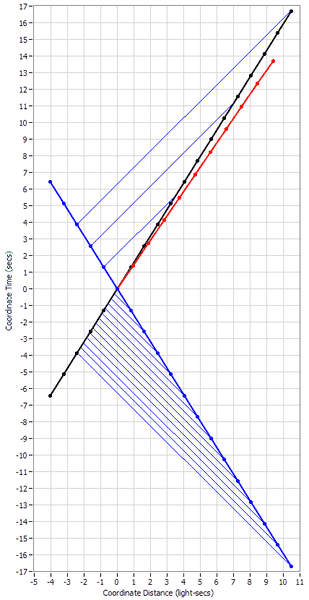Look at how the black body observes the ticking of the earth's clock. Starting from about 3 seconds before encounter, the black body sees the earth clock at 13 seconds before encounter. So the black body sees the earth's clock ticking about 13/3 or about 4.333 times faster than its own which is very close to the actual value of 4.359.

Then after encounter, the black body sees the earth's clock at 3 seconds when its own clock is at about 13 seconds. This is the inverse of the previous ratio or about 0.231 which is close to the actual value of 0.229.

I think in this frame you can easily see that if we drew in lines for how earth would observe the clock of the black body, it would be symmetrical. And if you remember from my previous post, it doesn't matter which frame we transform a scenario into, all observations of any observer remain the same. So we can say that the Doppler factors that each observer makes of the others clock both during approach and retreat are identical. This is an important consequence of the first postulate of Special Relativity, that in relative inertial motion, both observers will make the same observations of the other observer.

In this frame, the earth and the black body are both traveling at 0.6268c which determines a Time Dilation factor of 1.283 which neither observer can have any way to determine (because they don't know that we are looking at them in this frame) but if we use a frame where one of the observers is stationary, like the first one in my previous post then the Time Dilation factor at 0.9c is 2.294. There is a simple way that these two observers can calculate this value, they just average the two Doppler ratios that they determined, in this case (4.359/0.229)/2 = 4.588/2 = 2.294. But of course they would have to know this little trick.

Is there a way that they can calculate what the Time Dilation of the other ones clock would be in their own rest frame and without having to pass each other? Yes, there is, as I mentioned at the end of the previous post, using a combination of radar signals and observing the other ones clock. Here is a diagram showing the measurements that the earth makes of both the black body and the red body but in the black body's rest frame (just so we can't cheat):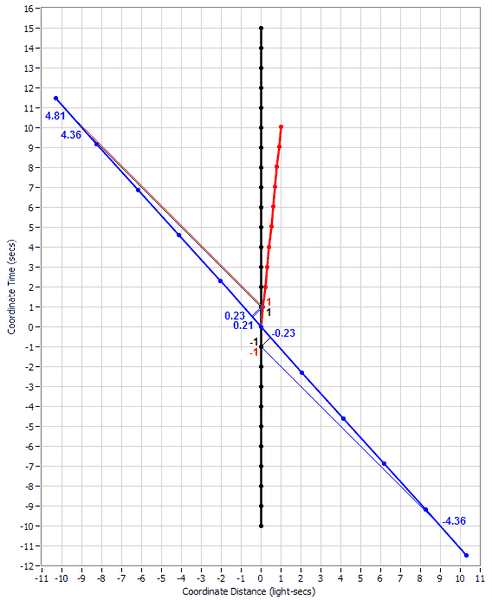Look first at the bottom of the diagram where the earth is approaching both bodies which start out at rest with respect to each other. Earth has been making continual radar measurements of the two bodies but I only show one significant set of radar signals. Earth sent out the signal at its time of -4.36 seconds and received the echo at -0.23. Remembering the calculations from post #8, this yields a measurement time of -2.295. (We don't care about the distance calculation.) Earth also observes that both clocks were at -1 second when the radar reflection occurred. These numbers are the result of the first measurement.

Now earth has to make a second measurement and for convenience, we will use the one at encounter where all the numbers are 0. (This same process will work for any other pair of measurements prior to encounter but it would take a larger diagram and twice as much calculation.)

So now earth can determine how much time would have progressed in its own rest frame during the time that the bodies progressed. It is 0-(-2.295) or 2.295 seconds and since the bodies progress through 1 second, the Time Dilation factor is 2.295, very close to the actual 2.294.

After the encounter, earth has to make two separate measurements for the two bodies since the red one accelerated at the encounter. For the black body, the measurements are 0.23 and 4.36 for an average of 2.295, the same as before encounter. No surprise there but let's do it for the red body. The numbers are 0.21 and 4.81 for an average of 2.51 and from post #5 we determined that the speed of the red body relative to earth is 0.917c which yields a Time Dilation factor of 2.507, a close match.

#### Attachments

Last edited:
•1 person
ViolentCorpse
I merely knew that relativistic doppler shift meant the frequency of light changes with relative motion. I had no idea it could affect time measurements as well.

I don't know how to thank you, ghwellsjr. Thank you so very much!

I wish I had teachers like you guys. :p

Last edited: